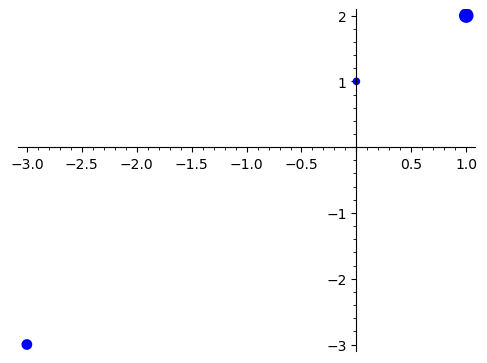# Plot points of different sizes

Say I have a map M:

[0,1] -> .3

[1,2] -> 1.1

[-3,-3] -> .6

etc.

I want to make a scatter plot where the point at P has size M[P]. How do I do this? Presumably I use the point2d object, which takes a size parameter.

edit retag close merge delete

Did you define M as a function? as a dictionary? Please provide the code you wrote so far.

Sort by » oldest newest most voted

Using point2d is indeed the right idea.

We will need to

• scale the values of M to turn them into reasonable point sizes for point2d.
• use a separate point2d command for each point size

Here is an example using the values in the question:

Define M as a dictionary:

sage: M = {(0, 1): 0.3, (1, 2): 1.1, (-3, -3): 0.6}


Plot:

sage: sum(point2d([p], size=100*s) for p, s in M.items())more